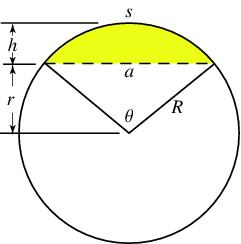# Diameter 5668

The span of the arc is 247 cm, and the height of the arc is 21.5 cm. What is the diameter of the circle?

D =  730.907 cm

### Step-by-step explanation:Did you find an error or inaccuracy? Feel free to write us. Thank you!

Tips for related online calculators
Are you looking for help with calculating roots of a quadratic equation?
Do you have a linear equation or system of equations and looking for its solution? Or do you have a quadratic equation?